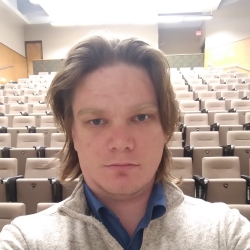# Stabilization of the response of cyclically loaded lattice spring models with plasticity

### Abstract

This paper develops an analytic framework to design both stress-controlled and displacement-controlled $T$-periodic loadings which make the quasistatic evolution of a one-dimensional network of elastoplastic springs converging to a unique periodic regime. The solution of such an evolution problem is a function $t\mapsto (e(t),p(t))$, where $e_i(t)$ is the elastic elongation and $p_i(t)$ is the relaxed length of spring $i$, defined on $[t_0,\infty)$ by the initial condition $(e(t_0),p(t_0))$. After we rigorously convert the problem into a Moreau sweeping process with a moving polyhedron $C(t)$ in a vector space $E$ of dimension $d,$ it becomes natural to expect (based on a result by Krejci) that the elastic component $t\mapsto e(t)$ always converges to a $T$-periodic function as $t\to\infty$. The achievement of this paper is in spotting a class of loadings where the Krejci’s limit doesn’t depend on the initial condition $(e(t_0),p(t_0))$ and so all the trajectories approach the same $T$-periodic regime. The proposed class of sweeping processes is the one for which the normals of any $d$ different facets of the moving polyhedron $C(t)$ are linearly independent. We further link this geometric condition to mechanical properties of the given network of springs. We discover that the normals of any $d$ different facets of the moving polyhedron $C(t)$ are linearly independent, if the number of displacement-controlled loadings is two less the number of nodes of the given network of springs and when the magnitude of the stress-controlled loading is sufficiently large (but admissible). The result can be viewed as an analogue of the high-gain control method for elastoplastic systems. In continuum theory of plasticity, the respective result is known as Frederick-Armstrong theorem.

Type
Publication
ESAIM: Control, Optimisation and Calculus of Variations 27 (2021) S8##### Ivan Gudoshnikov

My current research is on Moreau’s Sweeping process, its stability and appications.